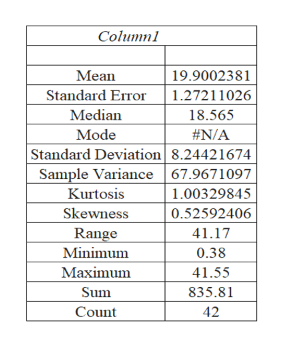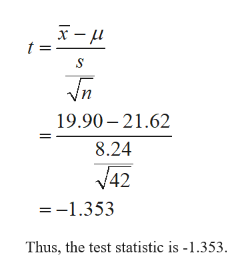# On its municipal website, the city of Tulsa states that the rate it charges per 5 CCF of residential water is \$21.62. How do the residential water rates of other U.S. public utilities compare to Tulsa's rate? The data shown below (\$) contains the rate per 5 CCF of residential water for 42 randomly selected U.S. cities.10.389.0811.76.412.3214.4315.4610.0214.416.0817.519.0817.8812.7516.717.2515.5414.718.8117.8914.818.3215.9526.7522.2222.6620.8823.3518.9523.619.1623.6527.726.9527.0426.8924.5837.7626.4138.9129.3641.55(a)Formulate hypotheses that can be used to determine whether the population mean rate per 5 CCF of residential water charged by U.S. public utilities differs from the \$21.62 rate charged by Tulsa. (Enter != for ≠ as needed.)H0:   Ha:   (b)What is the test statistic for your hypothesis test in part (a)? (Round your answer to three decimal places.) What is the p-value for your hypothesis test in part (a)? (Round your answer to four decimal places.) (d)Repeat the preceding hypothesis test using the critical value approach.State the null and alternative hypotheses. (Enter != for ≠ as needed.)H0:   Ha:   Find the value of the test statistic. (Round your answer to three decimal places.) State the critical values for the rejection rule. Use α = 0.05. (Round your answers to three decimal places. If the test is one-tailed, enter NONE for the unused tail.)test statistic≤test statistic≥

Question
96 views
On its municipal website, the city of Tulsa states that the rate it charges per 5 CCF of residential water is \$21.62. How do the residential water rates of other U.S. public utilities compare to Tulsa's rate? The data shown below (\$) contains the rate per 5 CCF of residential water for 42 randomly selected U.S. cities.
 10.38 9.08 11.7 6.4 12.32 14.43 15.46 10.02 14.4 16.08 17.5 19.08 17.88 12.75 16.7 17.25 15.54 14.7 18.81 17.89 14.8 18.32 15.95 26.75 22.22 22.66 20.88 23.35 18.95 23.6 19.16 23.65 27.7 26.95 27.04 26.89 24.58 37.76 26.41 38.91 29.36 41.55
(a)
Formulate hypotheses that can be used to determine whether the population mean rate per 5 CCF of residential water charged by U.S. public utilities differs from the \$21.62 rate charged by Tulsa. (Enter != for ≠ as needed.)
H0:

Ha:

(b)
What is the test statistic for your hypothesis test in part (a)? (Round your answer to three decimal places.)

What is the p-value for your hypothesis test in part (a)? (Round your answer to four decimal places.)

(d)
Repeat the preceding hypothesis test using the critical value approach.
State the null and alternative hypotheses. (Enter != for ≠ as needed.)
H0:

Ha:

Find the value of the test statistic. (Round your answer to three decimal places.)

State the critical values for the rejection rule. Use
α = 0.05.
(Round your answers to three decimal places. If the test is one-tailed, enter NONE for the unused tail.)
test statistic≤test statistic≥
check_circle

Step 1

(a)

The null and the alternative hypotheses are given below:

Step 2

(b)

Before calculating the test statistic find the mean and standard deviation,

Procedure:

• Enter the data into the Excel worksheet.
• Click Data, again click Data Analysis and then select Descriptive Statistics.
• Click and drag the entire range of the data for Input Range.
• Click on Grouped by Columns.
• Click on Summary Statistics.
• Click OK.

Output:help_outlineImage TranscriptioncloseColumn1 19.9002381 Mean Standard Error 1.27211026 Median 18.565 Mode #N/A Standard Deviation| 8.24421674 Sample Variance 67.9671097 Kurtosis 1.00329845 Skewness 0.52592406 Range 41.17 Minimum 0.38 Maximum 41.55 835.81 Sum Count 42 fullscreen
Step 3

Test statistic:

...help_outlineImage Transcriptioncloset = 19.90 – 21.62 8.24 V42 =-1.353 Thus, the test statistic is -1.353. fullscreen

### Want to see the full answer?

See Solution

#### Want to see this answer and more?

Solutions are written by subject experts who are available 24/7. Questions are typically answered within 1 hour.*

See Solution
*Response times may vary by subject and question.
Tagged in
MathStatistics

### Hypothesis Testing